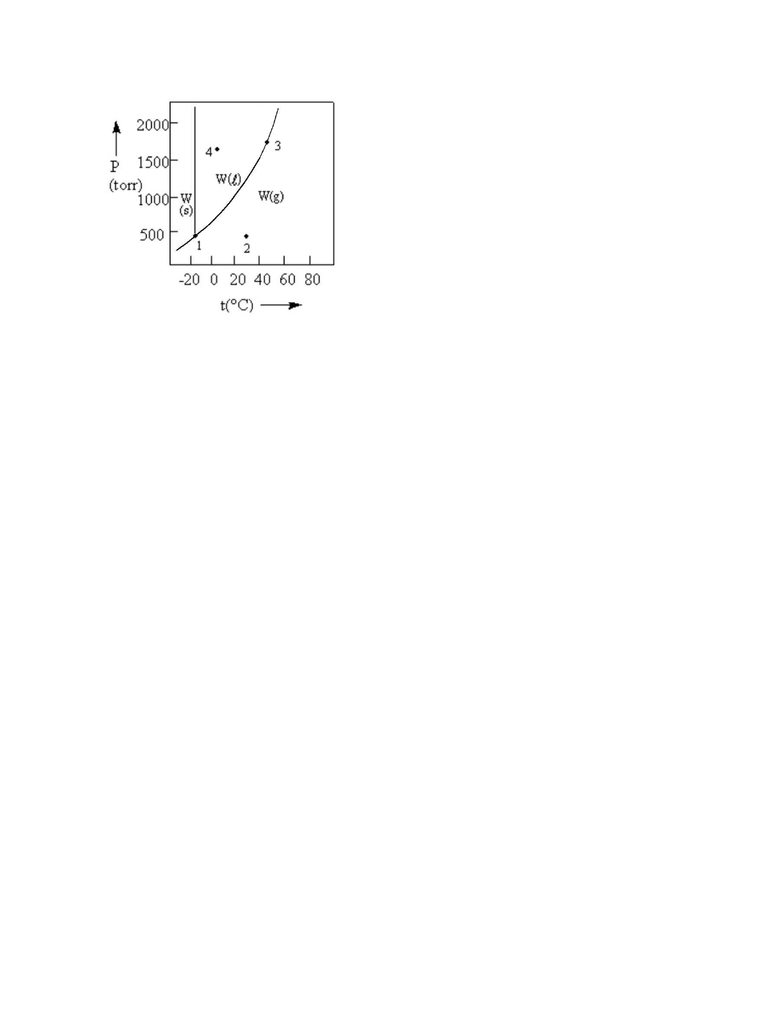# CHMA11_finalexam_2011.pdf

95 views11 pages
School
UTSC
Department
Biological Sciences
Course
BIOA02H3
ProfessorCHMA11 Final Exam, April 18 2011
Name___________________________________
MULTIPLE CHOICE. Choose the one alternative that best completes the statement or answers the question.
1)
What element is being oxidized in the following redox reaction?
MnO4 (aq) + H2C2O4(aq) Mn2+(aq) + CO2(g)
A)
H
B)
C
C)
D)
O
2)
Consider a reaction that has a positive ΔH and a positive ΔS. Which of the following statements is
TRUE?
A)
This reaction will be spontaneous only at low temperatures.
B)
This reaction will be nonspontaneous at all temperatures.
C)
This reaction will be nonspontaneous only at low temperatures.
D)
This reaction will be spontaneous at all temperatures.
E)
3)
Identify the location of oxidation in an electrochemical cell.
A)
the anode
B)
the socket
C)
the cathode
D)
the electrode
E)
the salt bridge
4)
Which of the pairs of molecules below have the same hybridization on the central atom in each
molecule? (The central atom is underlined.)
A)
Be
H
2
,
N
H
3
B)
H
2
O
, H
N
O
C)
H
O
Cl,
C
lF
3
D)
H
C
N,
C
O
2
E)
none of these
5)
Determine the [H 3O] in a 0.265 M HClO solution. The K a of HClO is 2.9 × 10
-
8
.
A)
7.7 × 10
-
9
M
B)
8.8 × 10
-
5
M
C)
4.9 × 10
-
4
M
D)
1.3 × 10
-
6
M
E)
1.1 × 10
-
10
M
6)
Identify the weakest acid.
A)
HI
B)
HF
C)
HBr
D)
HCl
E)
not enough information is available
#1
Unlock document

This preview shows pages 1-3 of the document.
Unlock all 11 pages and 3 million more documents.7)
pOH
=
3.14 is equivalent to:
A)
[H
+
] = 1.4 × 10
-
10
B)
pH
=
11.
C)
[OH
-
] = 7.2 × 10
-
4
D)
acidic solution
E)
none of these
8)
Which one of the following will form an acidic solution in water?
A)
NH
4
Cl
B)
NaF
C)
LiI
D)
KNO
3
E)
None of the above solutions will be acidic.
9)
Which of the following compounds solubility will not be affected by a low pH in solution?
A)
AgCl
B)
CuS
C)
BaCO
3
D)
CaF
2
E)
Mg(OH)
2
10)
Calculate ΔS°rxn for the following reaction. The S
for each species is shown below the reaction.
C2H2(g) + 2 H2(g) C2H6(g)
S°(J/mol·K) 200.9 130.7 229.2
A)
229.2 J/K
B)
-
102.4 J/K
C)
+303.3 J/K
D)
-
233.1 J/K
E)
+560.8 J/K
11)
Which of the following compounds is the most viscous:
CH3CH2OH
CH3CH2CH2CH3
HOCH2CH2OH
CH3OCH2CH3?
A)
There is not enough information.
B)
CH
3
CH
2
OH
C)
CH
3
OCH
2
CH
3
D)
CH
3
CH
2
CH
2
CH
3
E)
HOCH
2
CH
2
OH
2
Unlock document

This preview shows pages 1-3 of the document.
Unlock all 11 pages and 3 million more documents.12)
According to the phase diagram given, which of the following statements is FALSE?
A)
At the temperature of point 2, a pressure of 500 torr will cause W to liquefy.
B)
The existence of liquid W at
-
40
°
C and 500 torr represents the metastable condition of
"supercooling."
C)
At the temperature and pressure of point 1, W exists as a three
-
phase equilibrium system.
D)
If the system is maintained at the temperature of point 3 while pressure is decreased, more W will
vaporize.
E)
If W is maintained at the pressure of point 4 while the temperature is increased to 80
°
C, the liquid
will vaporize.
13)
Determine the molar solubility of CuCl in a solution containing 0.050 M KCl.
Ksp (CuCl) = 1.0 × 10-6.
A)
1.0 × 10
-
12
M
B)
1.0 × 10
-
3
M
C)
0.050 M
D)
2.0 × 10
-
5
M
E)
5.0 × 10
-
7
M
14)
When titrating a weak monoprotic acid with NaOH at 25°C, the
A)
pH will be greater than 7 at the equivalence point.
B)
pH will be equal to 7 at the equivalence point.
C)
titration will require more moles of acid than base to reach the equivalence point.
D)
titration will require more moles of base than acid to reach the equivalence point.
E)
pH will be less than 7 at the equivalence point.
15)
Determine the pH of a 0.18 M H 2CO3 solution. Carbonic acid is a diprotic acid whose K a1 = 4.3 × 10
-
7
and Ka2 = 5.6 × 10-11.
A)
3.56
B)
4.31
C)
11.00
D)
5.50
E)
10.44
3
Unlock document

This preview shows pages 1-3 of the document.
Unlock all 11 pages and 3 million more documents.

## Document Summary

Mno4 (aq) + h2c2o4(aq) mn2+(aq) + co2(g: h, c, mn, o, consider a reaction that has a positive h and a positive s. The s for each species is shown below the reaction. 130. 7: 229. 2 j/k, -102. 4 j/k, +303. 3 j/k, -233. 1 j/k, +560. 8 j/k, which of the following compounds is the most viscous: Ch3och2ch3: there is not enough information, ch3ch2oh, ch3och2ch3, ch3ch2ch2ch3, hoch2ch2oh. Carbonic acid is a diprotic acid whose k a1 = 4. 3 10-7 and ka2 = 5. 6 10-11: 3. 56, 4. 31, 11. 00, 5. 50, 10. 44. 3: balance the following redox reaction if it occurs in acidic solution. What are the coefficients in front of h. [baf2: use hess"s law to calculate g rxn using the following information. Clo(g) + o3(g) cl(g) + 2 o2(g) Sn(s) sn2+(aq, 1. 8 m)  ag+(aq, 0. 055 m) ag(s) Ni(s) ni2+(aq)  ag+(aq) ag(s: ag+(aq, ni2+(aq, ag(s, pt, ni(s) 3 fe(s) + 4 h2o(g)  fe3o4(s) + 4 h2(g)